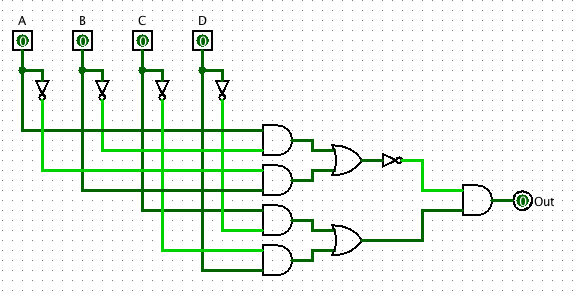# CS101 - Homework 6

Due: 2018-04-11 11:59p

#### Objectives

• Learn about truth tables, circuits and sum of products form

### [10 points] Prelab

Before coming to lab on Thursday / Friday, read this entire homework write-up and complete the prelab.

### [10 points] Reverse engineering

Below is a relatively simple circuit. It has four inputs (`A`, `B`, `C`, and `D`) and one output (`Out`). Your job is to reverse engineer this circuit and provide a single equation that describes its behavior.

The first step is to write the truth table. List out all possible combinations of 1 and 0 for the four variables. Then, for each pattern of 1s and 0s, trace through the logic and figure out if `Out` is a 1 or a 0.

Once the truth table has been constructed, list the minterms – all of the patterns where `Out` is equal to 1. OR them all together and you will have the sum of products form.

Note: You can use the symbol ~ ("tilde") to represent negation if you wish.### [10 points] A simple alarm

Design a simple alarm circuit. This circuit has four inputs: `SYSTEM_ON`, `DOOR`, `FLOOR`, and `WINDOW`. It also has one output: `ALARM`. The alarm should sound (be true), if the alarm system has been switched on and (at least) one of the sensors (`DOOR`, `WINDOW` `FLOOR`) is activated.

• Write a simple truth table for this function
• Use the truth table to help you devise a simple equation for when the alarm sounds
• Implement the circuit in Logisim. Note: Simplify! You won't want to create a circuit that implements the sum of products form of the full truth table. A much simpler circuit will work.

### Optional Challenge [up to 2 extra points]

This week's challenge is posted here.